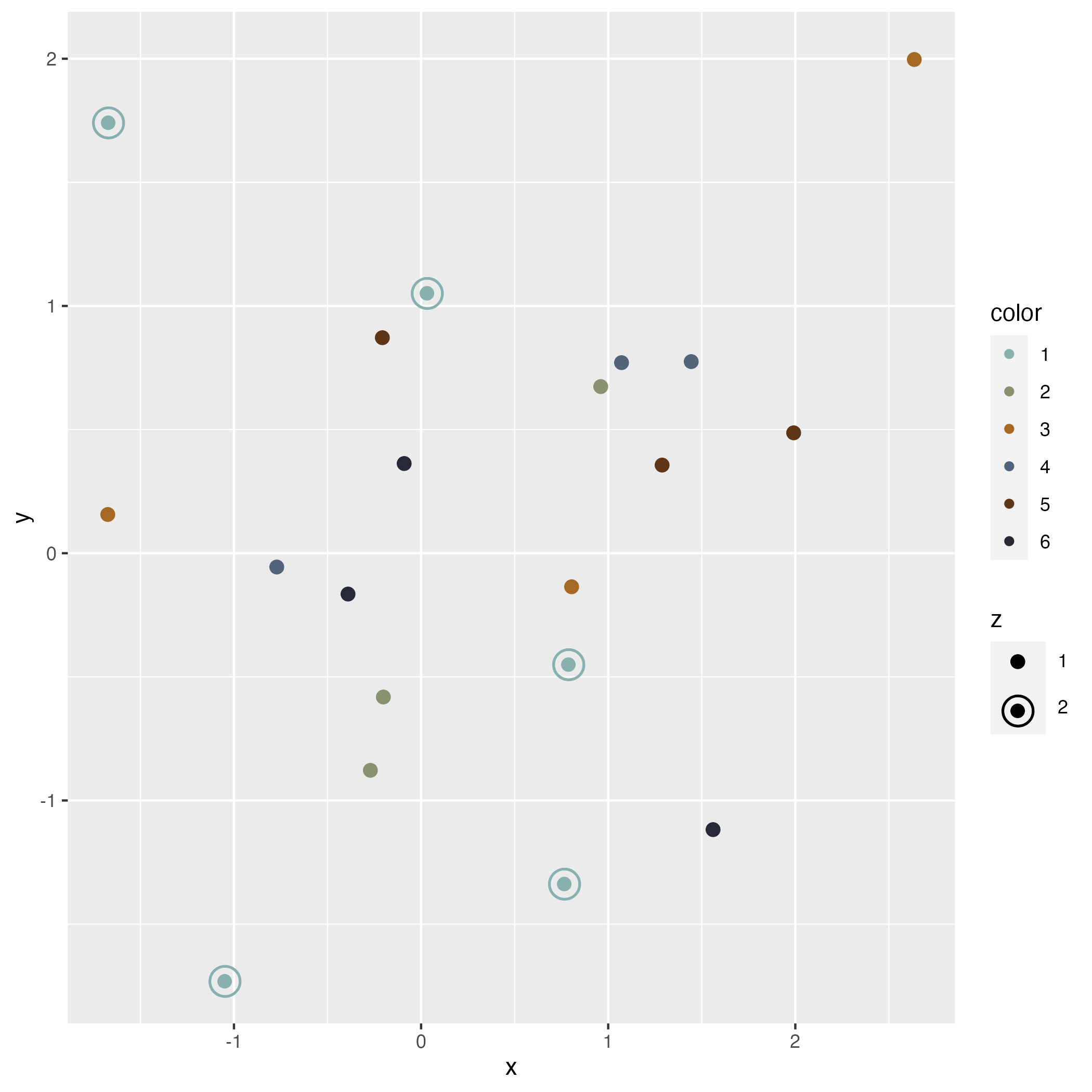## Installation

remotes::install_github("quartzsoftwarellc/ggquartz")

## Usage

scale_target() adds an alternating shape scale of a filled circle and a target and a alternating size scale for the size of the circles and targets. You can also use the bluegill_palette for added style.

library(magrittr)
library(ggplot2)
library(ggquartz)

data.frame(x = rnorm(20), y = rnorm(20), color = factor(c(rep(2:6, 3), rep(1, 5))), z= factor(c(rep(1, 15), rep(2, 5)))) %>%
ggplot(aes(x = x, y = y, shape = z, size = z, color = color)) +
scale_target() +
scale_color_manual(values = bluegill_palette) +
geom_point() -> p

ggsave("./example.png")plot